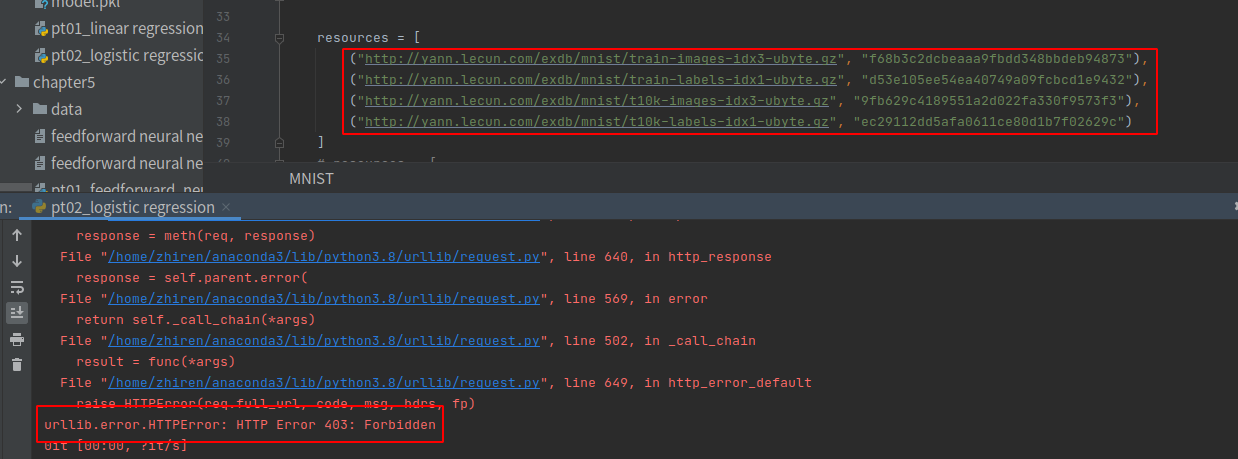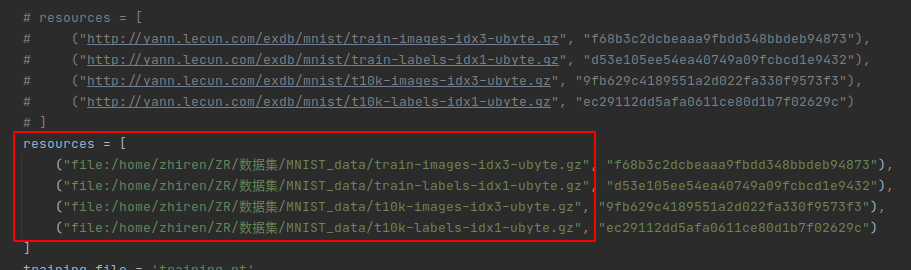• 1.一开始采用官网上利用input_data来加载本地数据集的方法，但会报出下面的错误 No module named 'tensorflow.examples.tutorials' 2.后面采用 keras加载mnist...解决办法：先将mnist数据集下载到本地，放到自己想...
注意：使用keras模块加载mnist数据集时，可以不用修改代码，直接将mnist数据集放到相应的路径下面。
windows: C:\Users\mac\.keras\datasets

1.一开始采用官网上利用input_data来加载本地数据集的方法，但会报出下面的错误
No module named 'tensorflow.examples.tutorials'

并且官网上input_data.py又下载不下来
解决方法：修改mnist.py（使用 ctrl + b，打开mnist.py），利用本地下载好的mnist数据集，直接将mnist.py里路径path改成本地mnist数据集的路径
下附代码：
main.py
from __future__ import absolute_import, division, print_function, unicode_literals
import tensorflow as tf

mnist = tf.keras.datasets.mnist

(x_train, y_train), (x_test, y_test) = mnist.load_data()
x_train, x_test = x_train / 255.0, x_test / 255.0

model = tf.keras.models.Sequential([
tf.keras.layers.Flatten(input_shape=(28, 28)),
tf.keras.layers.Dense(128, activation='relu'),
tf.keras.layers.Dropout(0.2),
tf.keras.layers.Dense(10, activation='softmax')
])

loss='sparse_categorical_crossentropy',
metrics=['accuracy'])

model.fit(x_train, y_train, epochs=5)

model.evaluate(x_test,  y_test, verbose=2)


mnist.py

"""MNIST handwritten digits dataset.
"""
from __future__ import absolute_import
from __future__ import division
from __future__ import print_function

import numpy as np

from tensorflow.python.keras.utils.data_utils import get_file
from tensorflow.python.util.tf_export import keras_export

Arguments:
path: path where to cache the dataset locally
(relative to ~/.keras/datasets).

Returns:
Tuple of Numpy arrays: (x_train, y_train), (x_test, y_test).

Yann LeCun and Corinna Cortes hold the copyright of MNIST dataset,
which is a derivative work from original NIST datasets.
MNIST dataset is made available under the terms of the
"""

path = "./mnist.npz"
x_train, y_train = f['x_train'], f['y_train']
x_test, y_test = f['x_test'], f['y_test']

return (x_train, y_train), (x_test, y_test)



展开全文数据集
• 下载MNIST数据集出现：urllib.error.HTTPError: HTTP Error 403: Forbidden，本地使用MNIST数据集 解决办法：将网页地址更换为本地地址 1.打开MNIST函数定义文件MNIST.py，定位到resource，分别复制resources中的四...
下载MNIST数据集出现：urllib.error.HTTPError: HTTP Error 403: Forbidden，本地使用MNIST数据集
解决办法：将网页地址更换为本地地址1.打开MNIST函数定义文件MNIST.py，定位到resource，分别复制resources中的四个网址，用浏览器打开并下载到制定文件夹，本文为：/home/zhiren/ZR/数据集/MNIST_data
（"http://yann.lecun.com/exdb/mnist/train-images-idx3-ubyte.gz",
("http://yann.lecun.com/exdb/mnist/train-labels-idx1-ubyte.gz",
("http://yann.lecun.com/exdb/mnist/t10k-images-idx3-ubyte.gz",
("http://yann.lecun.com/exdb/mnist/t10k-labels-idx1-ubyte.gz",

2.将源网址替换为本地地址，如下图所示。展开全文python pytorch
• 首先，下载mnist数据集到本地，下载地址：https://s3.amazonaws.com/img-datasets/mnist.npz 保存到一个文件夹中， 然后编码：(x_train, y_train), (x_test, y_test) = mnist.load_data(’/home/mayahong/NLP/...
首先，下载mnist数据集到本地，下载地址：https://s3.amazonaws.com/img-datasets/mnist.npz
保存到一个文件夹中，
然后编码：(x_train, y_train), (x_test, y_test) = mnist.load_data(’/home/mayahong/NLP/TensorFlow/tensorflow-101-master/mnist/mnist/mnist.npz’)
此路径为你刚刚保存的路径！
写相对路径(x_train, y_train), (x_test, y_test) = mnist.load_data(‘home/mayahong/NLP/TensorFlow/tensorflow-101-master/mnist/mnist/mnist.npz’)出错：
FileNotFoundError: [Errno 2] No such file or directory: ‘/home/mayahong/.keras/datasets/home/mayahong/NLP/TensorFlow/tensorflow-101-master/mnist/mnist/mnist.npz’


展开全文• MNIST数据集下载地址 https://github.com/amplab/datascience-sp14/raw/master/lab7/mldata/mnist-original.mat 首先在网上下载MNIST数据集，然后将下载的mnist-original.mat的数据放如程序的目录下 在代码中添加...
1、下载MNIST数据集下载地址
https://github.com/amplab/datascience-sp14/raw/master/lab7/mldata/mnist-original.mat

2、查看scikit数据根目录
from sklearn.datasets.base import get_data_home
print (get_data_home())
# C:\Users\lenovo\scikit_learn_data

3、在2中的文件目录下创建mldata文件夹，将下载的文件放到mldata文件夹中
4、在代码中加入如下代码：
mnist = fetch_mldata('MNIST original'）



展开全文python 机器学习
• python中Keras下载mnist数据集 方法一： 直接写代码进行加载数据集，如果没有下载数据集，它会自动进行下载。示例： from keras.datasets import mnist (X_train, y_train), (X_test, y_test) = mnist.load_data() ...
• Dataset之MNIST：自定义函数mnist.load_mnist根据网址下载mnist数据集(四个ubyte.gz格式数据集文件) 目录 下载结果 运行代码 下载结果 运行代码 mnist.py文件 # coding: utf-8 try: import ...
• 机器学习之sklearn使用下载MNIST数据集进行分类识别一、MNIST数据集1.MNIST数据集简介2.获取MNIST数据集二、训练一个二分类器1、随机梯度下降（SGD）分类器2、分类器的性能考核1.使用交叉验证测量精度2、混淆矩阵3、...机器学习 python
• 利用tensorflow2.0下载MNIST数据集 import tensorflow as tf data = tf.keras.datasets.mnist (train_x, train_y), (test_x, test_y) = data.load_data() 一般会出现如下报错: 原因是因为网络下载出错，解决方法是...python tensorflow
• scikit-learn使用fetch_mldata无法下载MNIST数据集的问题 0. 写在前面 参考书 《Python数据科学手册》 工具 python3.5.1，Jupyter Lab 1. 问题描述 如题，显示下载超时，链接不上，不能下载等。 2. 解决办法 直接...Python sklearn
• 1.自己下载mnist数据集下载地址：https://github.com/amplab/datascience-sp14/raw/master/lab7/mldata/mnist-original.mat 2.然后把这个.mat文件放到 ~/scikit_learn_data/mldata 文件夹 3.重新运行程序，...
• from sklearn.datasets import fetch_...scikit-learn中使用fetch_mldata下载MNIST数据集如果不成功可以采用下面的方法试试： 1. 直接从github上下载数据集，下载地址：https://github.com/amplab/datascience...python sklearn
• 利用Tensorflow训练mnist手写模型的代码，分享给大家可以自行下载tensorflow
• #运行会自动下载mnist数据集 mnist = input_data.read_data_sets("MNIST/", one_hot=True) ''' 但是存在一个问题： 原python文件里默认的下载地址是外网，没翻墙的话会一直下载不了 会提示： WARNING:tensorflow:...python tensorflow深度学习 人工智能
• 使用代码下载mnist数据集并且可视化部分图片代码实验结果 代码 #coding: utf-8 # 从tensorflow.examples.tutorials.mnist模块读取数据 from tensorflow.examples.tutorials.mnist import input_data # misc 是 ...
• 在tensorflow中用： from tensorflow.examples.tutorials.mnist import input_data mnist=input_data.read_...下载mnist数据集可能会出现url失效的情况，这种情况下可以替换tensorflow中的input_data脚本文件， 在这tensorflow
• 目录树结构： ...dataset中报错了下载mnist数据的代码，下载mnist数据以及缓存也会保存在这个文件中。 # coding: utf-8 try: import urllib.request except ImportError: raise ImportError('Yo...
• 首先需要用torchvision下载mnist数据集。 train_data = torchvision.datasets.MNIST( root='./mnist/', train=True, # this is training data transform=torchvision.transforms.ToTensor(),python 深度学习 卷积神经网络
• 直接使用下面代码下载数据集失败了 from tensorflow.examples.tutorials.mnist import input_data mnist = input_data.read_data_sets("MNIST_data/", one_hot=True) 解决方法 去网站手动下载 ...tensorflow
• mnist.py （功能，在线下载MNIST数据集） # coding: utf-8 try: import urllib.request except ImportError: raise ImportError('You should use Python 3.x') import os.path import gzip import pickle import...
• 看到一个大佬的解决方案，完美解决： https://blog.csdn.net/york1996/article/details/81780065pytorch
• 当我们导入mnist数据集的时候，很多教程告诉我们这样下载就可以： #coding=utf-8 import tensorflow as tf from tensorflow.examples.tutorials.mnist import input_data mnist = input_data.read_data_sets("MNIST_...
• tensorflow中 input_data.py加载mnist数据集 """ #SOURCE_URL = 'http://yann.lecun.com/exdb/mnist/' SOURCE_URL = 'mnist/' #离线下载数据集保存到mnist文件夹下 def maybe_download (filename, work_...tensorflow
• 1、MNIST数据集 2、训练一个二元分类器 2.1、随机梯度下降（SGD）分类器 2.2、分类器的性能考核： 2.2.1、混淆矩阵 2.2.2、精度:（我的理解：预测集内，预测正确的比率） 2.2.3、召回率：（我的理解：整个正类...sklearn
• 下载数据集之后，torchvision还会对数据集进行一些处理，将这些数据转换为.pt格式的文件，所以我们不能直接手动下载数据集然后放到相应的文件夹中，这会产生报错...【总结】不仅仅是mnist数据集，其他数据集下载速度过.pytorch...